Data Structures and Algorithms with Object-Oriented Design Patterns in C### A Simple Example

Consider the function f(n)=8n+128 shown in Figure. Clearly, f(n) is non-negative for all integers. We wish to show that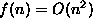. According to Definition, in order to show this we need to find an integer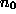and a constant c>0 such that for all integers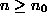,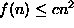.

It does not matter what the particular constants are--as long as they exist! For example, suppose we choose c=1. Then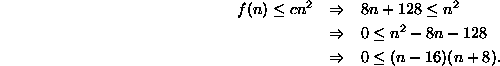Since (n+8)>0 for all values of, we conclude that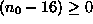. That is,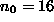.

So, we have that for c=1 and,for all integers. Hence,. Figureclearly shows that the function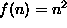is greater than the function f(n)=8n+128 to the right of n=16.

Of course, there are many other values of c andthat will do. For example, c=2 and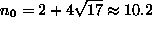will do, as will c=4 and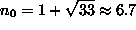. (See Figure).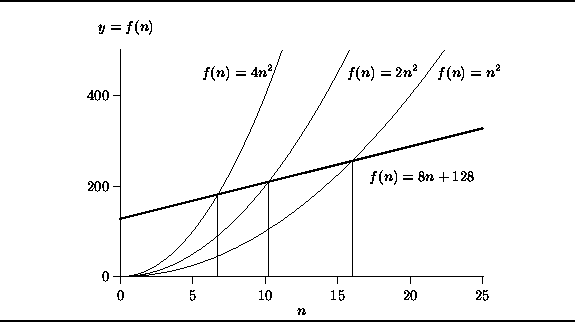Figure: Showing that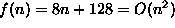.Copyright © 2001 by Bruno R. Preiss, P.Eng. All rights reserved.